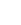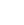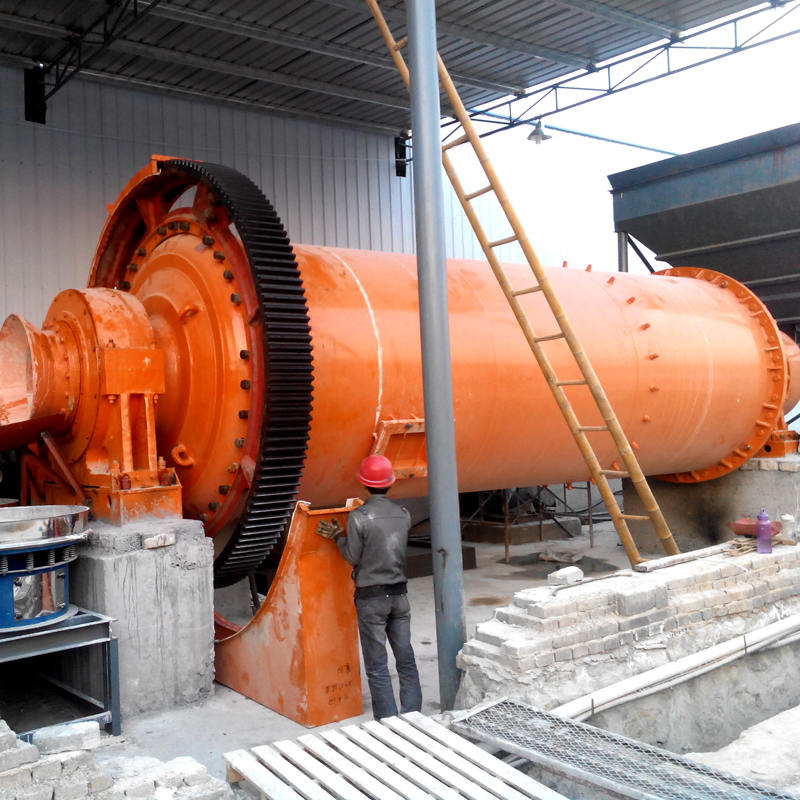•When Should We Add the Grinding Balls for Ball Mill
•sales@sinofote.comNews
Home > News > When Should We Add the Grinding Balls for Ball Mill# When Should We Add the Grinding Balls for Ball Mill

Dec 05,2017

When ball mill is rotating, grinding balls constant impact and rub with the grinding media, the material and the cylinder liner of ball mill. It not only causes abrasion of grinding balls but also lowers the grinding efficiency of ball mill. If the grinding ball can't be replenished in time, the ball mill's output and quality will decline.Therefore, we should add the grinding balls for ball mill timely in some cases. When should we add them? We can judge whether adding the grinding balls for ball mill according to four circumstances.

## 1. According to the unit time consumption of grinding balls

Under normal circumstances, we can calculate the average consumption in a unit time of grinding balls. Then we may make a monthly grinding ball adding plan according to the average consumption. In daily production, the grinding media can be added within this plan.

## 2. According to the current value reduce case of ball mill motor

In the normal operation of the ball mill, record the current value of ball mill motor. We can regard this as the corresponding current value of the rated grinding balls quantity. When the ball mill is running for a period of time, due to the abrasion of grinding balls, its quality will reduce and the current value of ball mill motor will also lower. At this point, we can properly replenish the grinding balls for ball mill, so that the current value can be restored to the value of the rated grinding ball number.

## 3. According to ball mill output and grinding balls consumption of unit product

The first thing is to do a good job of statistics. When replacing the grinding media each time, we should record separately the loading volume of the grinding balls in each silo and the amount of added grinding balls later. Then we can calculate out the grinding balls consumption of unit product according to the following formula.

E=(A+B-C)/M

E: grinding balls consumption of unit product

B: cumulative weight of regularly replenished grinding balls in the ball mill operation cycle (kg)

C: total weight of the grinding balls that is poured out during clearance (kg)

M: total weight of finished products in the ball mill operation cycle(t)

Through multiple statistical calculations, we can get the accurate grinding balls consumption of unit product. Then we can use the result times the daily output of ball mill, the daily consumption of the grinding balls can be obtained.

## 4. According to the flat surface reduction height of grinding balls in ball mill

⑴When measuring the inner sphere of ball mill, first we should stop feeding, and stop the ball mill running after 10 minutes.

⑵Next, we can open the mill gate and record the grinding balls surface height, and calculate the effective internal diameter of the ball mill.

⑶Then we can get the ratio of surface height and internal diameter.

⑷We can figure out the grinding balls filling rate according to the relationship between the ratio and the filling rate.